# HiSET Mathematics: Parallel Lines and Polygons Chapter Exam

Exam Instructions:

Choose your answers to the questions and click 'Next' to see the next set of questions. You can skip questions if you would like and come back to them later with the yellow "Go To First Skipped Question" button. When you have completed the practice exam, a green submit button will appear. Click it to see your results. Good luck!

### Page 1

#### Question 1 1. To prove the lines are parallel, if angle 3 measured 30 degrees, what must angle 6 measure?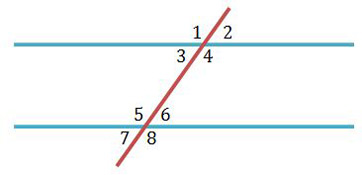#### Question 3 3. Which angle is equal to angle 1?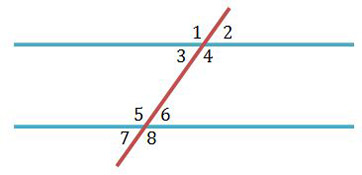#### Question 5 5. Which angle is the corresponding angle to angle 2?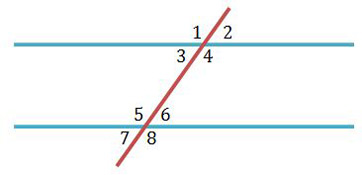### Page 2

#### Question 7 7. Which angle is the alternate exterior angle to angle 2?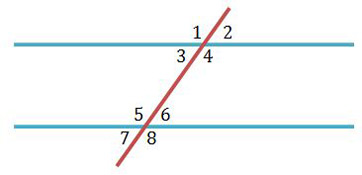#### Question 8 8. Find the perimeter (assume all sides are equal).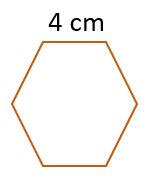#### Question 10 10. Name a pair of vertical angles.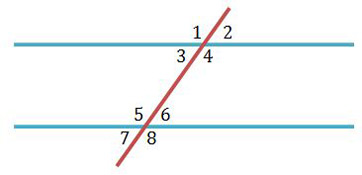### Page 3

#### Question 11 11. Which angles are supplementary if the lines are parallel?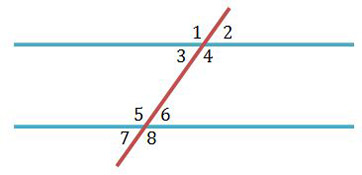#### Question 12 12. If angle 2 measures 60, what does angle 8 measure if the lines are parallel?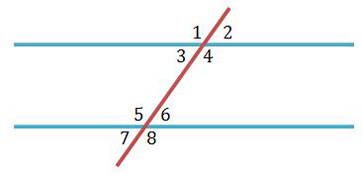### Page 4

#### Question 20 20. To prove the two lines are parallel, which pair of angles must be equal to each other if we used the converse statement regarding alternate exterior angles?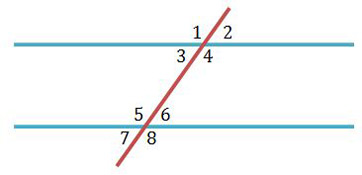### Page 5

#### Question 21 21. Find the perimeter.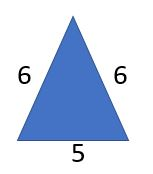#### Question 22 22. Name a pair of corresponding angles.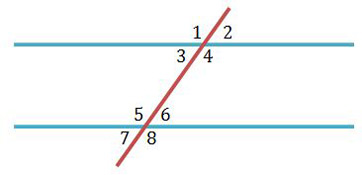#### Question 23 23. Which angle is the alternate interior angle to angle 3?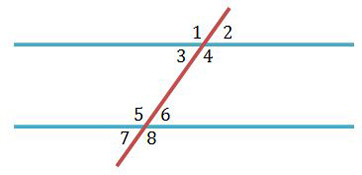#### Question 24 24. If angle 1 measures 120, what must angle 5 equal if the lines are parallel?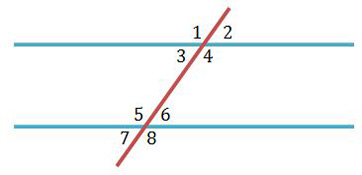#### Question 25 25. Find the perimeter.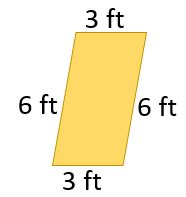### Page 6

#### Question 28 28. Find the perimeter.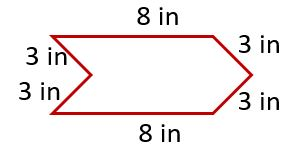#### HiSET Mathematics: Parallel Lines and Polygons Chapter Exam Instructions

Choose your answers to the questions and click 'Next' to see the next set of questions. You can skip questions if you would like and come back to them later with the yellow "Go To First Skipped Question" button. When you have completed the practice exam, a green submit button will appear. Click it to see your results. Good luck!

Support